Note: This is an archvied version of our old webpage. Some links might be broken. The current one can be found here.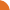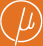Chair for Foundations of Software Reliability and Theoretical Computer Science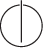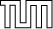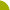Publications - On the Total Variation Distance of Labelled Markov ChainsReference:

Taolue Chen and Stefan Kiefer. On the total variation distance of labelled Markov chains. Technical report, arXiv.org, 2014. Available at http://arxiv.org/abs/1405.2852.

Abstract:

Labelled Markov chains (LMCs) are widely used in probabilistic verification, speech recognition, computational biology, and many other fields. Checking two LMCs for equivalence is a classical problem subject to extensive studies, while the total variation distance provides a natural measure for the ``inequivalence'' of two LMCs: it is the maximum difference between probabilities that the LMCs assign to the same event. In this paper we develop a theory of the total variation distance between two LMCs, with emphasis on the algorithmic aspects: (1) we provide a polynomial-time algorithm for determining whether two LMCs have distance 1, i.e., whether they can almost always be distinguished; (2) we provide an algorithm for approximating the distance with arbitrary precision; and (3) we show that the threshold problem, i.e., whether the distance exceeds a given threshold, is NP-hard and hard for the square-root-sum problem. We also make a connection between the total variation distance and Bernoulli convolutions.

Suggested BibTeX entry:

@techreport{14CK-LICS-TR,
author = {Taolue Chen and Stefan Kiefer},
institution = {arXiv.org},
note = {Available at http://arxiv.org/abs/1405.2852},
title = {On the Total Variation Distance of Labelled {M}arkov Chains},
year = {2014}
}See arxiv.org ...Conference version